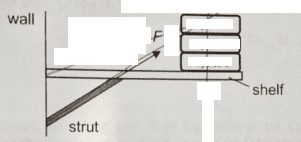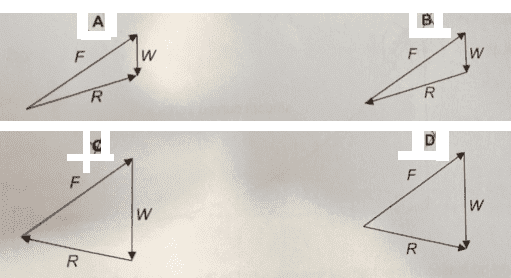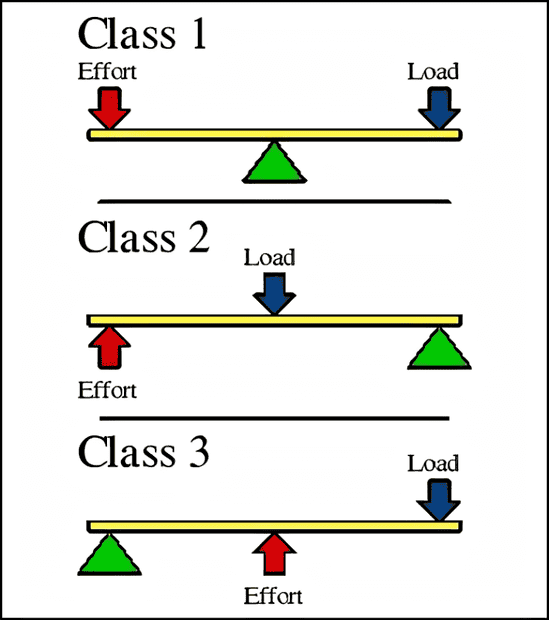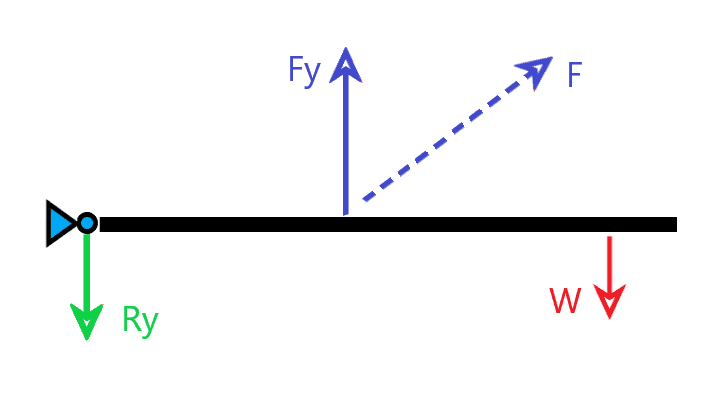# Force Diagram of Shelf

• songoku

#### songoku

Homework Statement
Figure below shows a light shelf supported by strut. The force by the strut is F, the force by the wall is R, and force by the books is W. Which diagram is correct?
Relevant Equations
Newton's LawJudging from FBD of the shelf, the answer will be either (B) or (D) because there should be horizontal force to the left to balance the horizontal force to the right by F, and by considering the torque, I think the answer will be (B)

But I am confused about the direction of the normal contact force between the shelf and wall. If the direction of R is like in (B), then the direction of the normal contact force will be to the left. This means that the wall exerts force on the shelf to the left, not to the right? I thought the force by the wall on the shelf will always be to the right.

Thanks

If you could replace the portion of the shelf that is located between the wall and its point of intersection with the strut with a spring, what do you believe it will happen?
The only horizontal component of force F points to the right and nothing in force W is doing anything to keep the static balance of forces; therefore the horizontal component of force R must do that.

Last edited:
•songoku
Thank you very much Lnewqban

•Lnewqban
In equilibrium, the net force is zero. That means the force polygon can be drawn with the forces in a nose to tail loop, as in B and C. A and D cannot be be so rearranged.
I'd say the harder part is ruling out C.

•songoku and Lnewqban
In equilibrium, the net force is zero. That means the force polygon can be drawn with the forces in a nose to tail loop, as in B and C. A and D cannot be be so rearranged.
I'd say the harder part is ruling out C.
Is it correct to rule out C by using torque (since in option C, R does pass the common point of W and F)?

Thanks

Is it correct to rule out C by using torque...
Just at a glance, we should be able to see that the magnitude of force F must be much greater than force W.
There are four reasons for that to be the case in this problem:

1) This configuration corresponds to a type 3 lever, for which the effort (vertical component of force F in this case) is always greater than the load.2) The connection of the shelf to the wall is unable to offer a resistive moment, and hence, the need for the supporting strut. Therefore, it can be considered a simple pivot.

3) The magnitude of force F must be greater than the vertical component of force F, due to the angle of the strut respect to vertical (both would be equal for the case of a vertical leg to the ground or a string supported from the ceiling).

4) In order to keep the balance of forces in the whole system, Rx must be equal in magnitude but opposite in direction to Fx, Ry must be equal in magnitude to Fy-W and pointing downwards. As a result, vector R should be pointing diagonally left and down, like in option B.•songoku
If three forces balance, they must intersect at a common point. Otherwise each has an unbalanced torque about the point of intersection of the other two.

By considering torque balance on the angled strut, F must act directly along the strut.
Hence the three forces intersect at a point above the shelf.

If the weight were to the left of where the strut and shelf meet, the intersection point would be below the shelf and C would be correct.

•songoku
I am really sorry for late reply.

Thank you very much for the help and explanation Lnewqban and haruspex

•Lnewqban